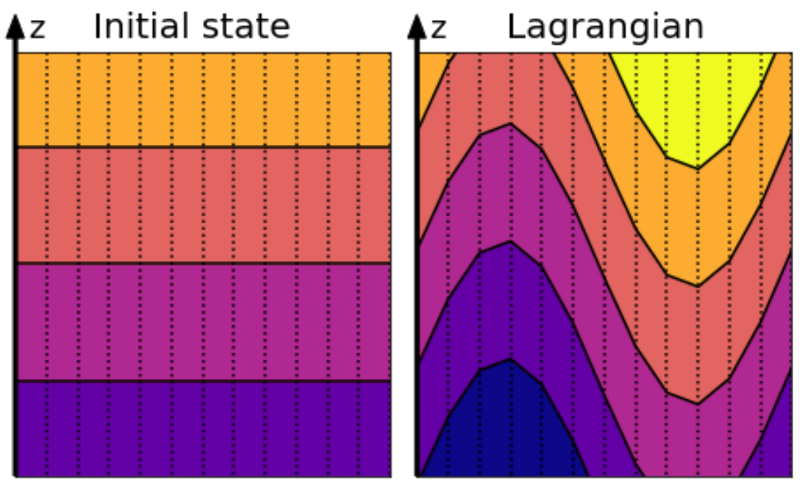# ALE Timestep¶

## Explanation of ALE remapping¶

The Arbitrary Lagrangian-Eulerian (ALE) remapping is not a timestep in the traditional sense, but rather an operation performed to bring the vertical coordinate back to the target specification. This remapping can be less frequent than the momentum or thermodynamic timesteps, but must be done before the layer interfaces become entangled with each other.

Assuming the target vertical grid is level $$z$$-surfaces, the initial state is shown on the left in the following figure:The initial state with level surface (left) and the perturbed state after a wave has come through (right).¶

Some time later, a wave has perturbed the surfaces which move with the fluid and it has been determined that a remapping operation is needed. The target vertical grid is still level $$z$$-surfaces, so this new target grid is shown overlaid on the left as regrid:

Mathematically, the new layer thicknesses, $$h_k$$, are computed and then populated with the new velocities and tracers:
$h_k^{\mbox{new}} = \nabla_k z_{\mbox{coord}}$
$\sum h_k^{\mbox{new}} = \sum h_k^{\mbox{old}}$
$\vec{u}_k^{\mbox{new}} = \frac{1}{h_k} \int_{z_{k + \frac{1}{2}}}^{z_{k + \frac{1}{2}} + h_k} \vec{u}^{\mbox{old}}(z')dz'$
$\theta^{\mbox{new}} = \frac{1}{h_k} \int_{z_{k + \frac{1}{2}}}^{z_{k + \frac{1}{2}} + h_k} \theta^{\mbox{old}}(z')dz'$